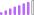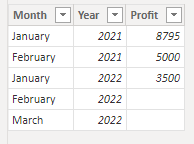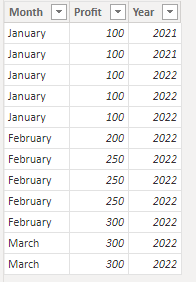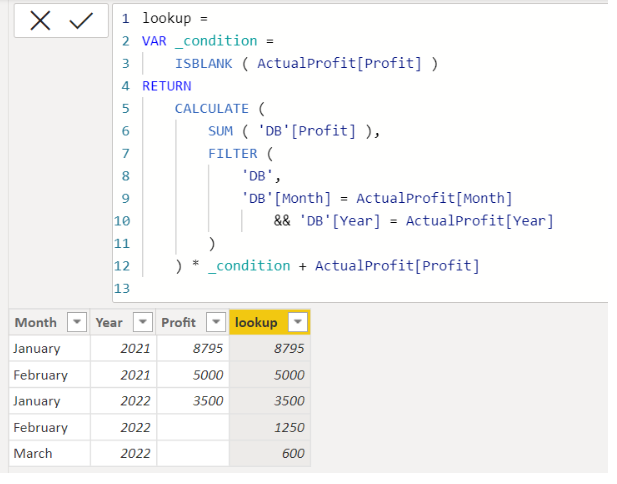cancel
Showing results for
Did you mean:Post Partisan

## LOOKUPVALUE

Dear Community,

As I have two tables, DB and ActualProfit, I would like to look up the sum of DB table based on month and year into ActualProfit table, but Do not replace the numbers in Actual Profit that are already filled up.This is Actual Profit table.This is DB table.

I would like to sum the value of months February and March in the DB table then look into the Actual Profit table.
Knowing that this code can look up the total profit, but will replace the actual profit that has been filled

`lookup = CALCULATE(SUM('DB'[Profit]),FILTER('DB','DB'[Month]= ActualProfit[Month] && 'DB'[Year] = ActualProfit[Year] ))`Expectation:Any help will be greatly appreciated.

1 ACCEPTED SOLUTIONSuper User

Hey @NickProp28 ,

You can try something like this:

``lookup = if(ActualProfit[Profit]<> BLANK(),ActualProfit[Profit],CALCULATE(SUM('DB'[Profit]),FILTER('DB','DB'[Month]= ActualProfit[Month] && 'DB'[Year] = ActualProfit[Year] )))``

This will create a new column taking values from the Profit if they are already there and if not, it will do the calculation and fill the result. The outcome will be as:Will this solve the purpose?

2 REPLIES 2Super User

Hi,

Please check the below picture and the attached pbix file.

It is for creating a new column.``````lookup =
VAR _condition =
ISBLANK ( ActualProfit[Profit] )
RETURN
CALCULATE (
SUM ( 'DB'[Profit] ),
FILTER (
'DB',
'DB'[Month] = ActualProfit[Month]
&& 'DB'[Year] = ActualProfit[Year]
)
) * _condition + ActualProfit[Profit]
``````

If this post helps, then please consider accepting it as the solution to help other members find it faster, and give a big thumbs up.Super User

Hey @NickProp28 ,

You can try something like this:

``lookup = if(ActualProfit[Profit]<> BLANK(),ActualProfit[Profit],CALCULATE(SUM('DB'[Profit]),FILTER('DB','DB'[Month]= ActualProfit[Month] && 'DB'[Year] = ActualProfit[Year] )))``

This will create a new column taking values from the Profit if they are already there and if not, it will do the calculation and fill the result. The outcome will be as:Will this solve the purpose?